We will now move into the important topic of Platonic solid nesting and transitions.

In essence, the Platonic solids are not five separate shapes, but five aspects of the same shape (the spinning sphere/torus.)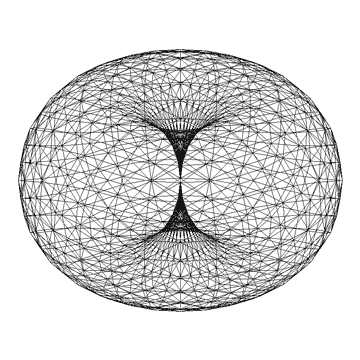When one Platonic solid is present, they are all present.  They cannot be separated.  They arise together as one – each in potentiation within the torus.  Certain shapes physically manifest depending on the nature of the manifestation (scale, function, type of life form…etc.).

We saw in the previous article how the Archimedean solids come directly from the Platonic solids.  We also saw how the Platonic solids can be stellated, or joined in various compounds to form other solids.

This is the key idea: – every solid can transition into any other solid through a series of movements including twisting, truncating, expanding, combining, or faceting.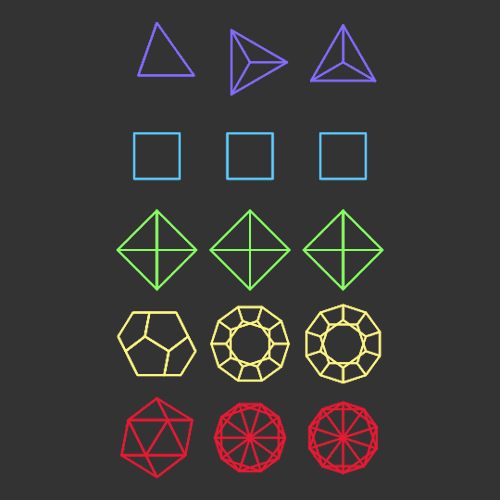We will begin by discussing Johannes Kepler and nested Platonic solids.  We will then show several examples of Platonic solid transitions.

It is important to note that these solids can transform one into the other in many, many ways.  We will only cover a few here.

These Platonic solid transitions harken back to Plato and his Timaeus.  He speaks abundantly on the ability of the four elements to ‘transmute into each other’ and how ‘they must be dissimilar to one another, but capable of arising out of one another’s disintegration.

“In other words, it looks as though there’s a cyclic process whereby they generate one another.” 49c. “They are changing even while they’re being identified.” 50b

We are referring to the four elements as four of the Platonic solids: the tetrahedron as fire; the octahedron as air; the cube as earth and the icosahedron as water.

He writes in 32b, “Hence between fire and earth the god placed water and air, and he made them all stand in the same ratio to one another (in so far as that is possible), so that as fire is to air, so air is to water, and as air is to water, so water is to earth, and so he bound together and structured the visible and tangible universe.”

Later in Timaeus Plato writes, “the variety of ways [the Platonic solids] can interact, either just with themselves or with one another, is infinite.”  57d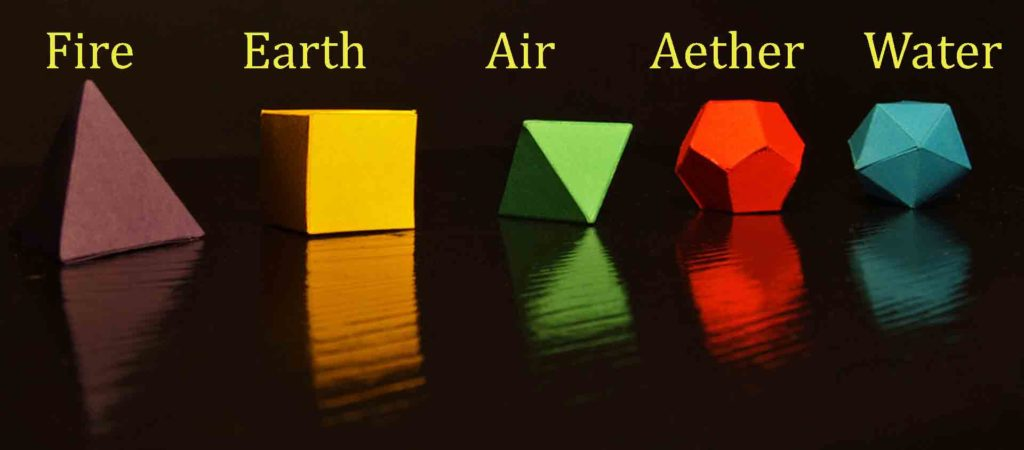Kepler and the Platonic Solids

Johannes Kepler (1571-1630) was a famous German mathematician, astronomer and astrologer.  He is a key figure in the 17th century scientific revolution.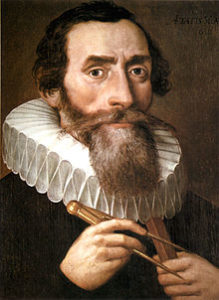He said, “I much prefer the sharpest criticism of a single intelligent man to the thoughtless approval of the masses.”

Kepler’s version of the solar system was as one Platonic solid within another, the radii of the intervening concentric spheres relating to the orbits of the planets.

Kepler “found that each of the five Platonic solids could be inscribed and circumscribed by spherical orbs; nesting these solids, each encased in a sphere, within one another would produce six layers, corresponding to the six known planets – Mercury, Venus, Earth, Mars, Jupiter, and Saturn.  By ordering the solids selectively – octahedron, icosahedron, dodecahedron, tetrahedron, cube – Kepler found that the spheres could be placed at intervals corresponding to the relative sizes of each planet’s path, assuming the planets circle the Sun.”1

His nested Platonic solids include an octahedron at the center, then an icosahedron, then dodecahedron, then tetrahedron, then cube.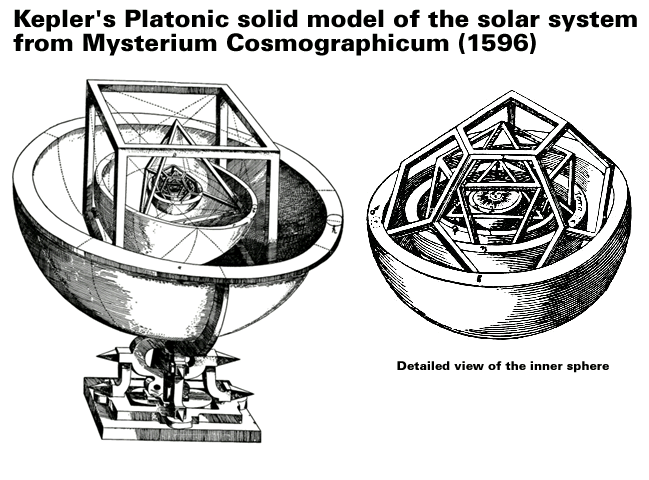Kepler also found a formula relating the size of the each planet’s orb to the length of its orbital period.  He later rejected this formula because it was not accurate enough.

Kepler said, “Where there is matter, there is geometry.”

And “Geometry is the archetype of the beauty of the world.”However, Kepler never relinquished the Platonist polyhedral-spherist cosmology that he published in his Mysterium Cosmographicum in 1596.

He later turned his attention to chronology and ‘harmony’, the numerological relationships among music, mathematics and the physical world, and their astrological consequences.  “By assuming the Earth to possess a soul he established a speculative system connecting astrological aspects and astronomical distances to weather and other earthly phenomena.”2

Kepler published Harmonices Mundi in 1619.  He was convinced “that the geometrical things have provided the Creator with the model for decorating the whole world.”3

He attempted to explain the proportions of the natural world – particularly the astronomical and astrological aspects – in terms of music.

Kepler later developed the three laws of planetary motion after using Tycho Brahe’s extensive astronomical data.

Tycho Brahe (1546-1601) was a Danish nobleman, astronomer, astrologer and alchemist.  His astrological observations were five times more accurate than the best available at the time.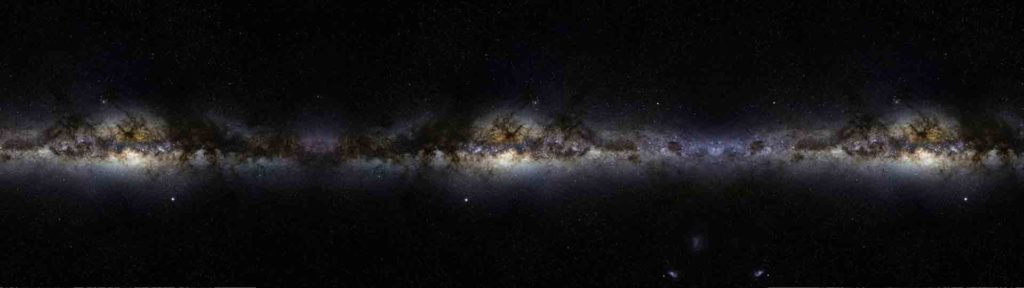Nested Platonic Solids

All Platonic solids nest within each other.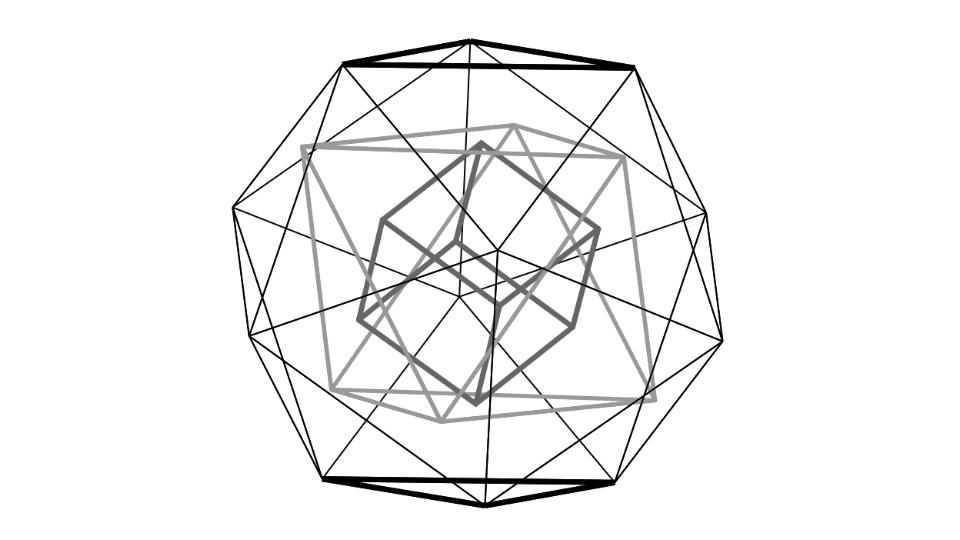Remember, they are not five separate shapes, but five aspects of the same shape (the spinning sphere/torus).

When one Platonic solid is present, they are all present.  They cannot be separated.  They arise together as one – each in potentiation, with certain shapes physically manifesting depending on the nature of the manifestation (scale, function, type of life form…etc.).

As we said, there are many ways in which these solids nest and transform one to the other.  We already saw how Platonic solid duals can transition one into the other.  We will discuss several other ways now.

In the next several articles we will focus on one solid at a time where we will look at some of these transitions in greater detail.

The Nested Platonic Solids

See page 154-155 and 376 in the Quadrivium.

Each solid fits together in various ways.

A cube fits within a dodecahedron.

A cube has 12 edges.  The cube will have one edge along each of the twelve faces of the dodecahedron, on which the edge of the cube is a diagonal of a pentagon face of the dodecahedron.  (The diagonal of a pentagon would be one of the five lines forming the pentagram of the pentagon.)

Each of the colored lines below represents a different cube found in the dodecahedron.  There are five total:  red, yellow, green, blue, and black.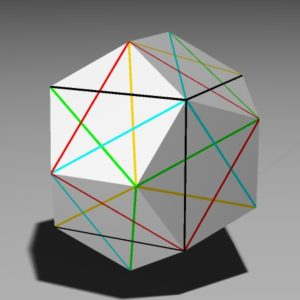You can also see how one can build a dodecahedron by adding 6 ‘roof’ shapes (pictured below) to the 6 faces of a cube.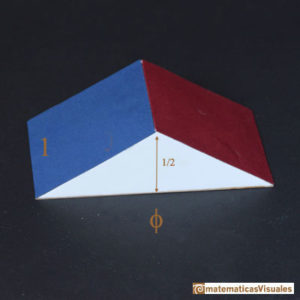dodecahedron side = 1

cube side length = phi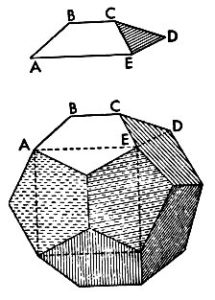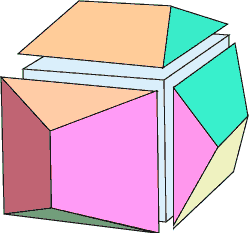What this means is that the dodecahedron can turn inside out to form a cube, or vice versa.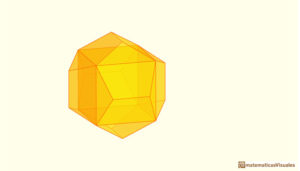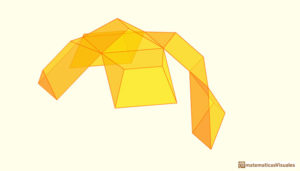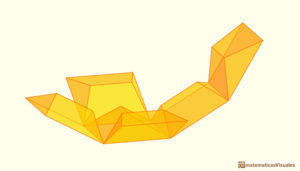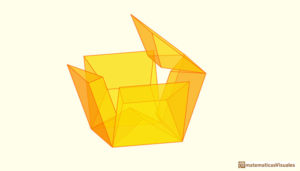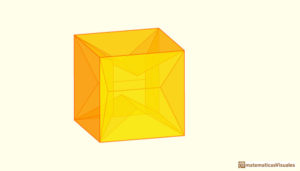Credit: Matematicas Visuales – http://www.matematicasvisuales.com/english/index.html

Visit Matematicas Visuales for great visual geometry!  Many thanks for their work!

A tetrahedron nests within the dodecahedron & cube.

A tetrahedron can be inscribed in a cube.  Each of the 6 edges of the tetrahedron shows up as one of the diagonals of one of the 6 faces of the cube.  The 4 four vertices of the tetrahedron share 4 of 8 vertices of the cube.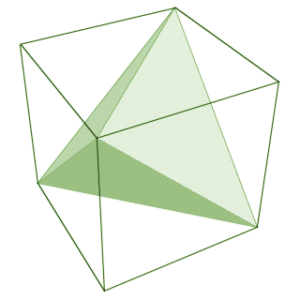Tetrahedron side length = √2 x cube edge length

The tetrahedron = 1/3 the cube’s volume or .33333…

A tetrahedron fits into the dodecahedron as well.

The 4 vertices of the tetrahedron can be oriented and scaled to match exactly with 4 of the 20 vertices of the dodecahedron.  This means five tetrahedra can fit inside a dodecahedron.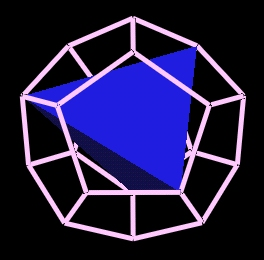Below is an image of the compound of 5 tetrahedra.  If you connect the top points of each color you draw a pentagon.  This would be one of the 12 faces of the dodecahedron.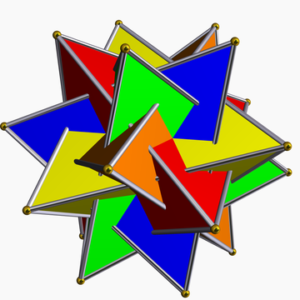See http://www.rwgrayprojects.com/Java3D/Dodeca/Dodeca.html for illustrations of all 5.

An octahedron nests within a tetrahedron.

The 6 edge midpoints of the tetrahedron define the 6 vertices of the octahedron.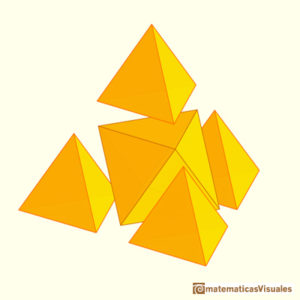The octahedron halves the tetrahedron’s edges.

The octahedron halves the tetrahedron’s surface area & volume.

This embodies the octave 1:2.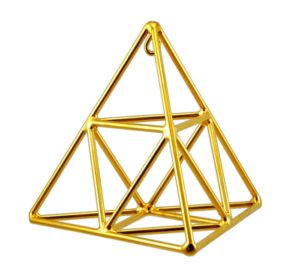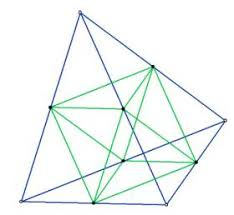An icosahedron nests within an octahedron.

Each of the 12 edges of the octahedron correspond to the 12 vertices of the icosahedron.

The icosahedron’s vertices cut the octahedron’s edges perfectly in the Golden Section.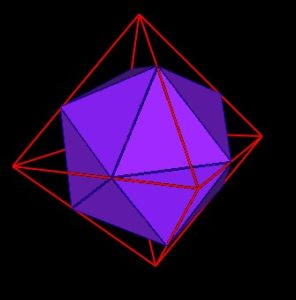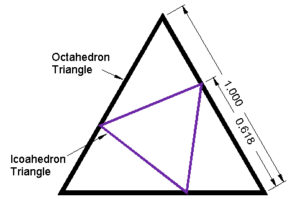It is interesting to note here that the octahedron and icosahedron are different phases of the Jitterbug motion.  Additionally, the 8 triangles of the Jitterbug pass through a regular dodecahedron phase.

“If the position of one of the Jitterbug triangles in the dodecahedron position is drawn in the triangular face of an octahedron, we get the following diagram:”4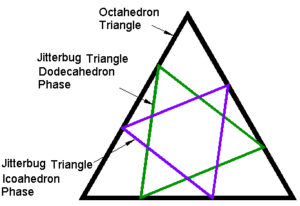All five can thus be nested within each other in the following way:

Icosahedron within an Octahedron within a tetrahedron within a cube within a dodecahedron…within an icosahedron…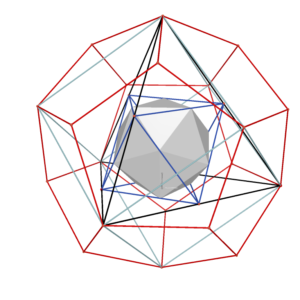This can continue to infinity in both directions.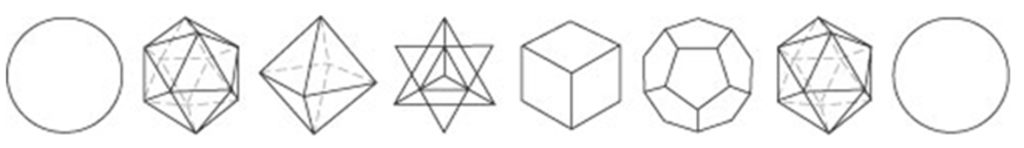The Tetrahedron as ‘Master’ Solid

The tetrahedron is at the apex and center, being the master solid.  This drawing below by Keith Critchlow in Order in Space shows the hierarchy and relationship of the five regular solids and their spherical counterparts to the tetractys, a triangular pattern made up of ten units.  This tetraktys was the basis of Pythagorean harmony.

The whole tetractys pattern can be given tetrahedral form, in which case the figurate number will be twenty not ten.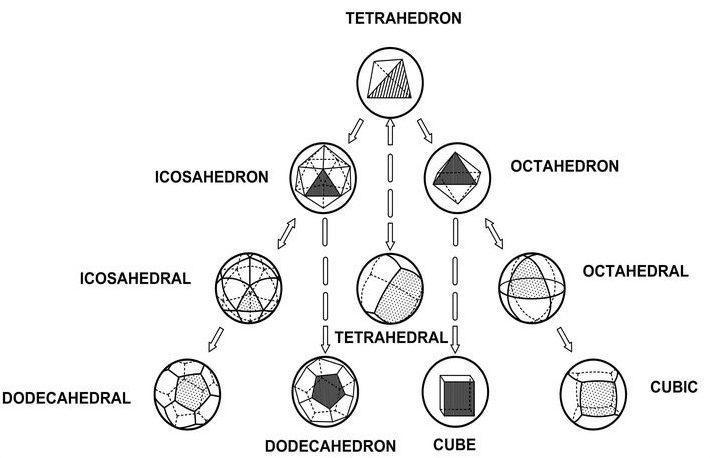All Platonic Solids come from the Tetrahedron

Here are some ways:

Tetrahedron and Octahedron

Note – tetrahedrons do not appear in nature alone, but always with their dual.

The dual tetrahedron (star tetrahedron) contains within it an octahedron.

The star tetrahedron itself is a stellated octahedron (stella octangula).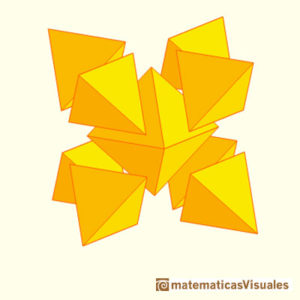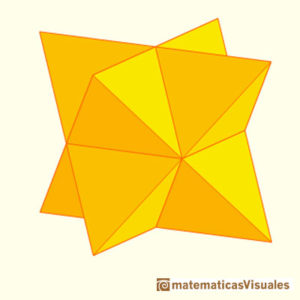Additionally, the tetrahedron can be deeply truncated (rectification) to form the octahedron.

Removing a tetrahedron of half the original edge length from each vertex of the tetrahedron produces the octahedron.

The vertices of the octahedron lie at the midpoints of the edges of the tetrahedron.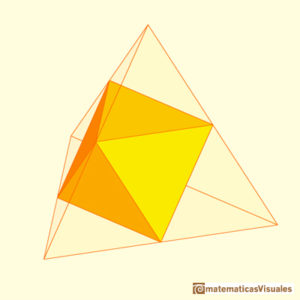Tetrahedron and Icosahedron

Note – it takes 20 tetrahedrons to form an icosahedron.  Each face of the icosahedron would be connected to the centroid (center point of the icosahedron).

However, the tetrahedrons would have to be compressed somewhat to form a perfect icosahedron.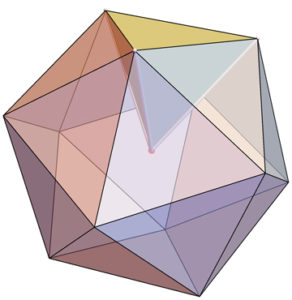If each icosahedron edge is 1 unit long, then the tetrahedron edges incident to the centroid (center point of the icosahedron) have length:

½ [√½ (5+√5)] ≈ 0.95

The tetrahedra would be slightly non-regular when constructed in this way.5

Octahedron and Icosahedron

As we saw above, if one divides the edges of a regular octahedron in an appropriate way using the golden section, it can be shown that the twelve division points are the vertices of a regular icosahedron.

Three golden rectangles (in 3 mutually orthogonal planes) make an icosahedron.  “These rectangles have 12 vertices.  The distance between any pair of neighboring points is equal to the short side of one golden rectangle.  These 12 points coincide with the 12 vertices of an icosahedron.”6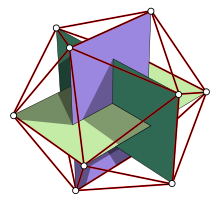Here is the icosahedron inside a cube using three pairs of equal, parallel and mutually orthogonal edges of the icosahedron.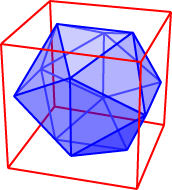“Since each of these golden rectangles can be easily inscribed in a (diagonally placed) square, we find a nice way of placing an icosahedron inside an octahedron…The rectangles are inscribed in the three determining squares of the octahedron.  The vertices of the golden rectangles divide the edges of the octahedron in the golden ratio φ:1.”7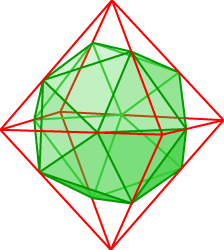Dodecahedron and Cube

Note – eight of the vertices of a regular dodecahedron correspond to the 8 vertices of a cube.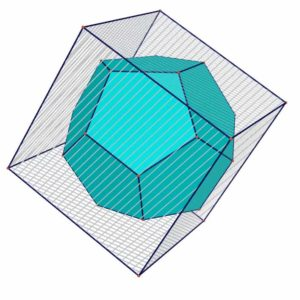In this cube we inscribe a regular tetrahedron.  The midpoints of the edges of this tetrahedron are the vertices of a regular octahedron.

In this octahedron we construct a regular icosahedron using the method explained before.

In this way we obtain a very nice combination of the five regular polyhedra.

This is the icosahedron inside the octahedron inside the tetrahedron inside the cube inside the dodecahedron.The following diagram shows, once again, that the tetrahedron is the most basic of the platonic solids.  It may be considered the fundamental platonic shape since all platonic and Archimedean solids can be constructed by mathematical functions operating over this shape.8

Cant – to bevel a face at its edges and vertices

Snub – an expansion with the faces moved apart, and twists on their centers, adding new polygons centered on the original vertices, and pairs of triangles fitting between the original edges.

Truncate – to cut off corners to create equal edge lengths

Rectification – complete truncation – edges are reduced to points.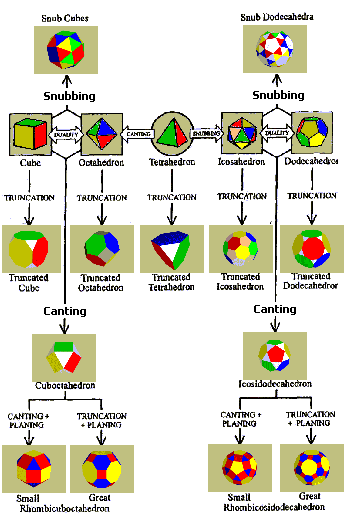The Genesis of the Platonic Solids (Cosmic Volumes)

All of the Platonic solids have a nesting relationship that is embodied in the golden section.

Starting with the Icosahedron, it grows by an additive and geometric process simultaneously based upon the golden section.

Reference Construction Lesson #41: The Genesis of the Platonic Solids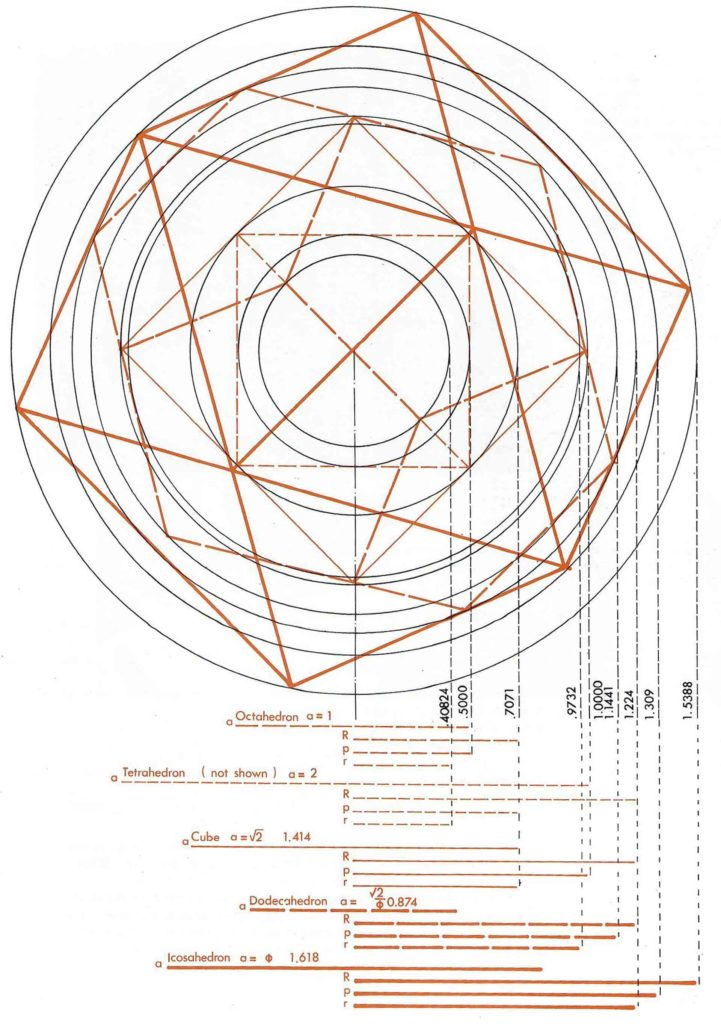Credit: Robert Lawlor – Sacred Geometry: Philosophy & Practice

The Icosahedron into the Dodecahedron

It can be seen that through φ, the ‘divine seed’, the icosahedron takes form.

The generation of the dodecahedron arises spontaneously, a result of the natural crossing of all the internal radiants of the icosahedron.  These two figures are the inverse of one another: both are composed of 30 edges, but whereas the icosahedron has 20 faces and 12 vertices, the dodecahedron has 12 faces and 20 vertices.“If we connect all the internal vertices of the icosahedron by drawing three lines from each one connecting it to the ones opposite, and then from the two upper vertices draw four lines to the opposite ones, and allow these lines to converge at the center, we will in so doing form naturally the edges of the dodecahedron.  This is a generation which happens by itself through the crossing of the internal radiants of the icosahedron.”9

The Dodecahedron into the Cube

“Once we have established the dodecahedron we can, simply by using six of its points and the center, form a cube.”10The establishment of the dodecahedron automatically gives rise to the cube defined by the 8 vertices of the dodecahedron, the edges coinciding with one diagonal of each face.The Cube into the Star Tetrahedron

“Simply by using the diagonal of the cube we are able to form the star-tetrahedron or interlocking tetrahedron.”11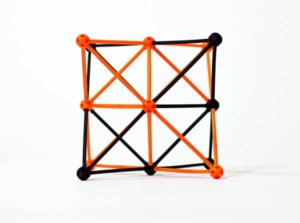The diagonals of the faces of this cube form an interlocking star tetrahedron.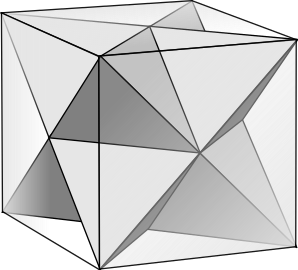The cube perfectly contains a star tetrahedron.  The octahedron, like the cube, is seen in 2D perspective as a hexagon.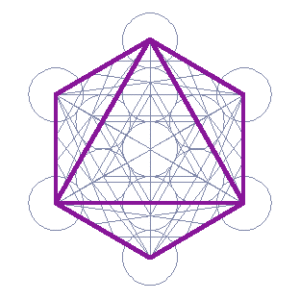The octahedron seen in 2D as a hexagon.

Only the dodecahedron is not contained by the outline of the hexagon.

The Star Tetrahedron into the Octahedron

“The intersections of the star-tetrahedron with the cube give us the perfect positioning to form an inscribed octahedron.”12The volume enclosed by the two interlocking tetrahedra defines an octahedron, thus completing the composite group of regular polyhedra.

The Octahedron to the Icosahedron

“Within the octahedron, using again the lines given by the internal radiants of the icosahedron, along with the points of the octahedron, there arises a second icosahedron.We have gone through a complete cycle, through five stages, from seed to seed.  “This then is an infinite progression.”13

Side length of Cube = 1

Side length of outer Icosahedron = φ

Side length of dodecahedron = 1/φ

Side length of star tetrahedron = √2

Side length of octahedron = 1/√2

Side length of inner Icosahedron = 1/φ2 (0.382…)

Sequence of the Platonic Solids

This sequence comes from the work of artist and geometrician Frank Chester.

Fully truncating (rectifying) the tetrahedron creates the octahedron.  (Discussed above.)The octahedron’s points are truncated to form the cube.  Cube & Octahedron are duals.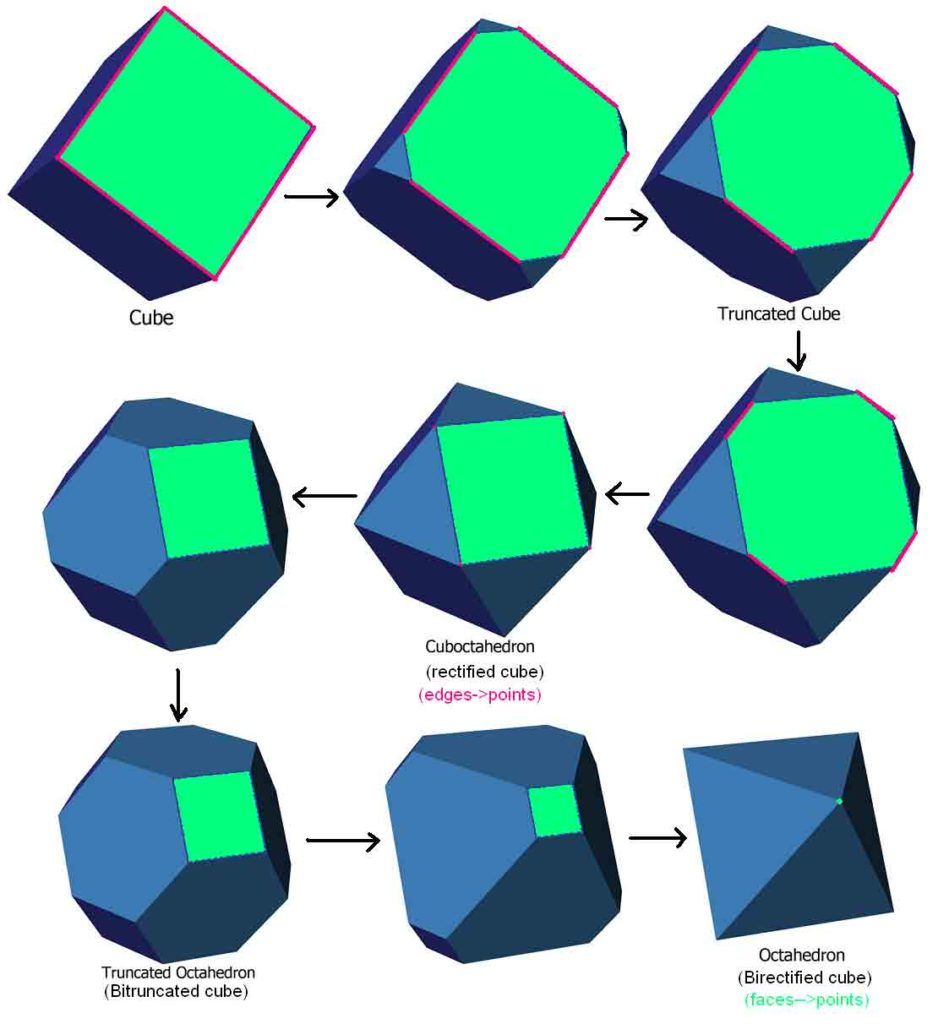The cube transforms into the dodecahedron if the edges of the cube are pushed into planes.  Here the edges are truncated, instead of corners truncated.  This is called cantellation.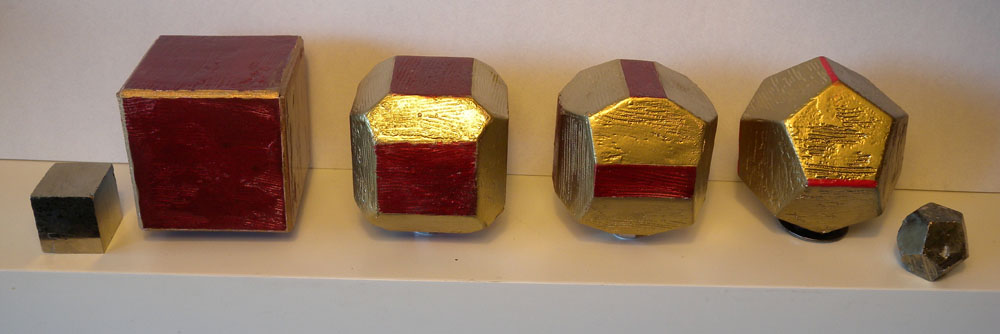Then the dodecahedron’s points are truncated to yield the icosahedron.  The dodecahedron and icosahedron are duals.Hence: tetrahedron – octahedron – cube – dodecahedron – icosahedron

Different orderings have been traditionally used, but Frank’s sequence is the first based on phenomenological, not abstract or concept-based, transformations of the forms.

The Chestahedron as a Balanced Form of Rest amidst the Platonic Solid Transformations

The Chestahedron – the 7-sided figure discovered by Frank Chester – is discussed in Article 67.

It is the first known seven-sided solid with faces of equal area.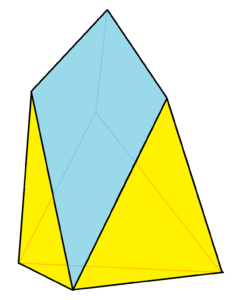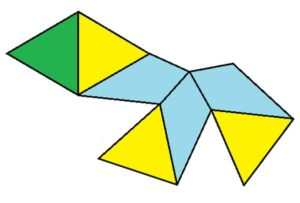It has: 7 Faces; 7 Vertices; 12 Edges.

Its Faces are 7 in total.  That is, 4 triangles and 3 kite-shaped quadrilaterals.

Its Vertices are 7 in total.  That is, 4 have 3 edges meeting and 3 have 4 edges meeting.

Its Dual is the Dekatria, a 13-sided figure.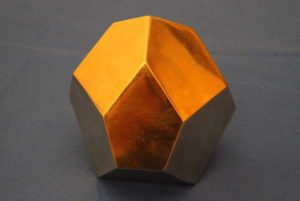The Chestahedron can be formed through a vortex motion of the dodecahedron, tetrahedron and octahedron:

The Pentagonal Face of the Dodecahedron:

The two-dimensional, unfolded version of the Chestahedron relates exactly to a perfect five-pointed star (pentagram).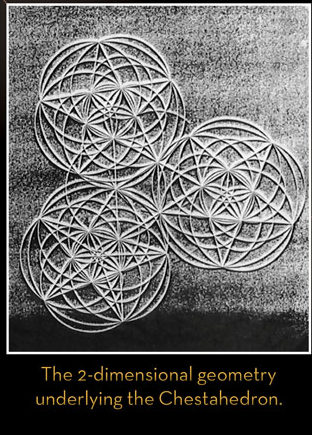Credit: Frank Chester

Five of the kite shapes, placed with their points together, make the star pentagon.

This means the Chestahedron can be created by folding up a star pentagon on itself, bringing it into three dimensions.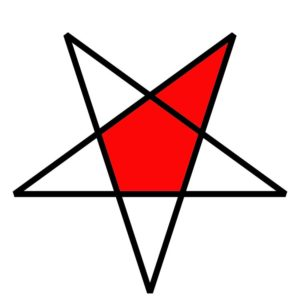The blue faces of the Chestahedron shown in the net above correspond to the center and one point of a pentagram.

It also relates to the icosahedron and dodecahedron.

The fundamental uniting factor is the base equilateral triangle.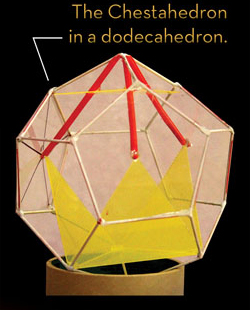Credit: Frank Chester

The Chestahedron can be created in Two Distinct Ways from a Tetrahedron:

First way: Contractive

Place a tetrahedron inside a cube, and while keeping the boundaries of the cube inviolate, twist the tetrahedron so that one of its points moves along the diagonal of one square face to the opposite corner.

The tetrahedron, at this point, has changed into the octahedron.There are actually 2 moments between the transformation of the tetrahedron into the octahedron that result in the chestahedron.

The geometry of the Chestahedron is a geometry of motion (vortex motion).

The Chestahedron itself is merely a balanced moment of rest in a whole field of geometric activity that involves all of the Platonic forms.

Second way: Expansive

This begins with the tetrahedron, which is then unfolded like a flower with three petals.

See this in detail at: www.frankchester.com/2009/chestahedron-from-a-tetrahedron/.

The opening of the tetrahedron immediately creates the seven-sided form with the addition of the three kite-shaped faces.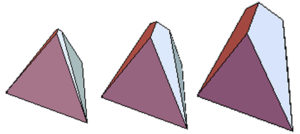It only remains to unfold the petals to the exact angle at which the area of the kite faces equals the area of the equilateral triangles at angle 94.83°.This unfolding sequence, traced through time, can be taken further.

If the unfolding of the petals continues past the point at which the chestahedron arises, a moment comes when the dihedral angle becomes 109.47°.

At this moment it takes the shape of a perfect octahedron with a tetrahedron on top that is exactly the size of the original tetrahedron.

This whole form itself is bounded by a tetrahedron that is exactly twice the size of the original.

As we have been examining all throughout Cosmic Core, all in life and reality is based upon a geometric matrix that is made up of the regular polygons, five Platonic Solids and 13 Archimedean solids, as well as all the various truncations, stellations, combinations and transition states of these forms.

The Chestahedron is a perfect example of what geometry can be formed from through a transitional movement of the Platonic solids.

It is helpful to remember that ‘All is Motion’.   So, even though we think of geometry as static figures and forms, in reality, geometry is continually pulsing, oscillating, spiraling and transforming from one shape to another to another, and so on…Geometric Energy Transitions

The following sequence was discussed by David Wilcock on his website: https://divinecosmos.com/.

Sphere to Icosahedron

The sphere into the icosahedron represents the movement of formless Unity into geometric form.

Icosahedron to Octahedron

By angularly tilting the icosahedron on its side (we have not calculated the exact number of degrees of tilt that are necessary,) and adding a special harmonic tetrahedral shape in twelve different places, we can build the octahedron.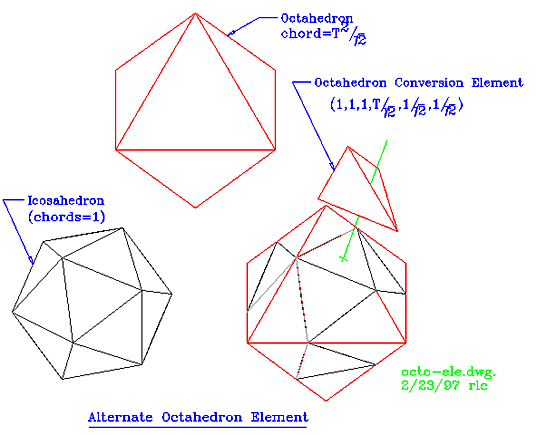Octahedron to Star Tetrahedron

Expand each face of the octahedron into a basic four-sided triangle, or tetrahedron.Each face on the octahedron has eight sides.  Add eight tetrahedra to its faces.  If animated, it would appear that the octahedron was suddenly blooming like a flower; the faces suddenly sprout upwards as the tetrahedra rise into position.

As we saw above, the space in the center of a star tetrahedron is an octahedron – so you are putting a tetrahedron on each of the 8 faces of the octahedron to get the star tetrahedron.

It is important to remember at this point that these harmonic shapes do not simply “sit there” in space-time – they are oscillating and rotating.

The spherical torus that surrounds them shows us where the axis of rotation is. Notice that if the octahedron would normally rotate on an axis that went from tip to tip, it would be forced to tilt at a 45-degree angle to its side as it becomes the star tetrahedron, which then would have a different tip-to-tip axis.

Star Tetrahedron to Cube

If we connect the tips of the star tetrahedron together we will get a cube.

This can be seen in the above diagram, where the hexagonal “box” formation is drawn around the six outer tips of the star tetrahedron.

Cube to Dodecahedron

A further outward expansion is required, where each face of the cube sprouts a slanting “rooftop” made of five equidistant lines in order to turn into the dodecahedron. (Discussed above.)For this expansion, the cube must tilt into one of two different angular positions (which we have not calculated) as the dodecahedra’s own rotational axis is established.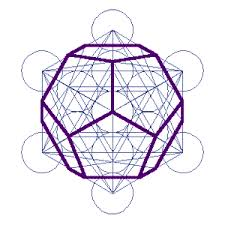Dodecahedron to Icosahedron

If you put a dot in the center of each pentagon on the dodecahedron and connect all of the dots together, you will have a series of lines that form five-pointed stars that create the icosahedron shape, the last major node before the return to the Sphere.Each line in the new icosahedron divides each line of the dodecahedron exactly in half because they are duals.  No rotational tilt of the axis is necessary to complete this transition.

The below diagram, created on Bruce Rawle’s Sacred Geometry website shows the Platonic solids interpenetrating with their duals.  The dodecahedron into an icosahedron is shown on the far right.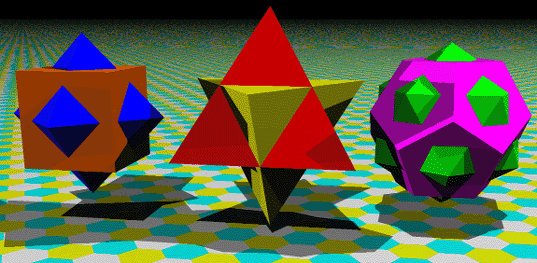Icosahedron to Sphere

In this Octave system, the icosahedron is the first geometry to crystallize out of the sphere and the final geometry to exist before the vibrations melt back into the purity of the sphere once again.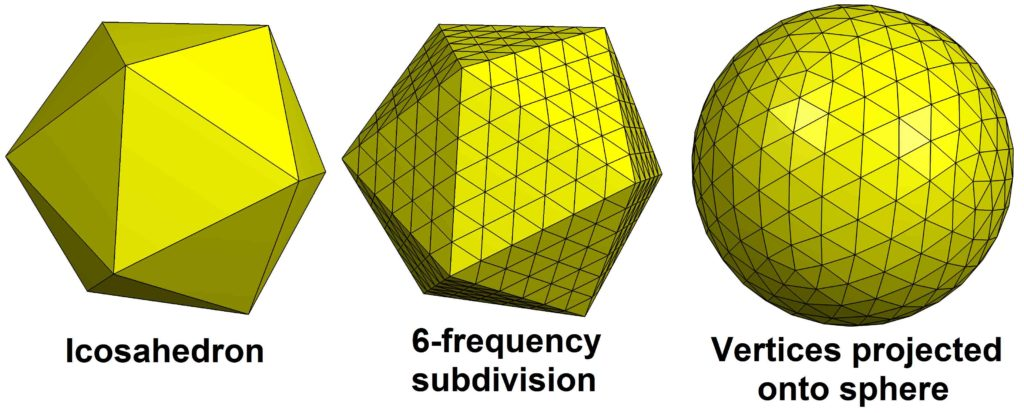In any of these cases where the Platonic Solids are seen to expand, a ratcheting, tilting motion must take place as the spiral causes the shape to naturally expand.

In short, going back to our original harmonic table again, we can see how the entire progression is a sphere, or a Oneness, expanding into the ‘seed’ or fundamental form of the icosahedron, which then by its structure gives rise to all of the other forms contained therein.14

When you have a higher vibration (higher frequency, shorter wavelength), the shapes transform into objects of greater complexity.  This is illustrated through Cymatics.  See Article 121.

What we have here is an understanding of the fact that the shapes formed by these energy vibrations can grow, much in the way that crystals grow.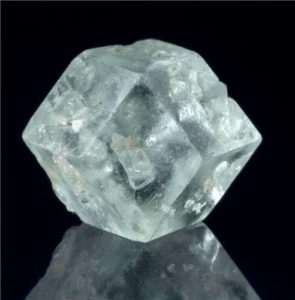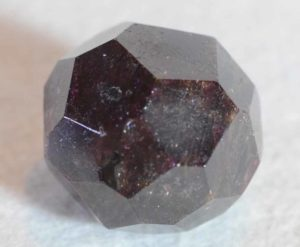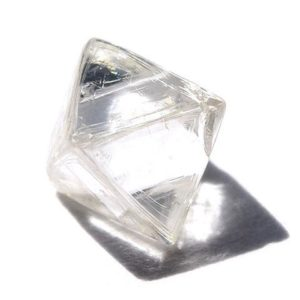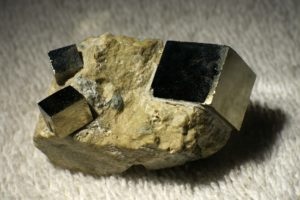Buckminster Fuller’s Cosmic Hierarchy

We will now cover one more transition of the Platonic Solids – that of Buckminster Fuller’s Jitterbug.

Bucky Fuller (1895-1983) was an American architect, system theorist, author, designer and inventor.  He is famous for the geodesic dome design and construction.

He called himself a “comprehensivist”, that is, one who holds a comprehensive perspective that seeks to synthesize apparent “parts” into an ever-unifying holistic understanding.

His stated mission:  “to create a world that works for 100% of humanity through spontaneous cooperation and without ecological offense or the disadvantage of anyone.”Fuller’s cosmic hierarchy is related to the way the Platonic solids nest within each other.  His version is one of the many sequences of how the Platonic solids transition from one to the other.

Fuller’s cosmic hierarchy starts with the star tetrahedron (the tetrahedron and its dual).  The star tetrahedron pulses outwards to form the cube and its dual the octahedron.  From this come the icosahedron and its dual the dodecahedron.  Then comes the vector equilibrium and its dual, the rhombic dodecahedron.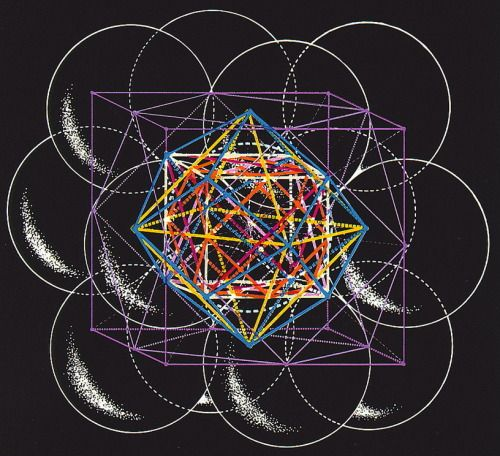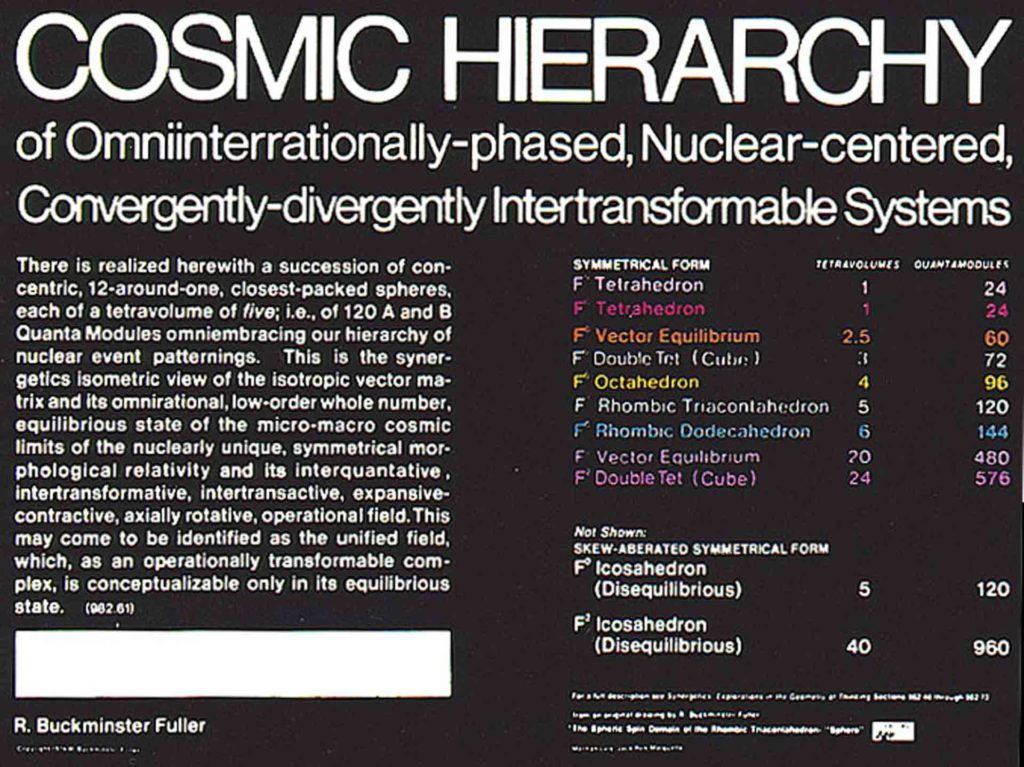Jitterbugging

Buckminster Fuller’s explanation of ‘jitterbugging’ once again relates to the nesting properties of Platonic solids.  The jitterbugging motion is a result of the cuboctahedron’s (vector equilibrium’s) ability to transform into each and every Platonic solid.  The vector equilibrium is the ground state geometry of the Aether.

The jitterbugging exhibits a pulsating dynamic that arises when it moves out of equilibrium and returns back again.  During its non-equilibrium phase it manifests the primary structural forms of the Platonic solids.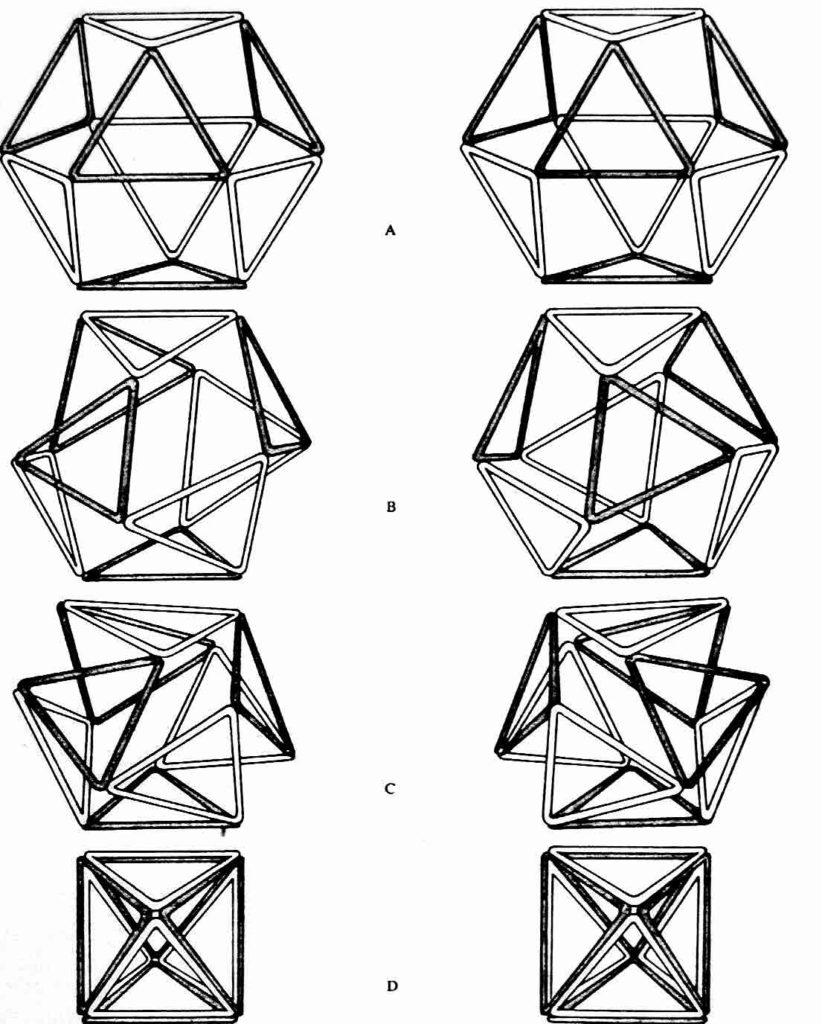The vector equilibrium (VE) has square and triangular faces.

The square faces are unstable structurally – this allows for the VE to collapse in a spiraling motion (jitterbugging).

It can rapidly collapse and expand in both left and right spirals, pulsating and oscillating like a dancer.

As it does, it transforms through phases that include symmetrical articulation of the icosahedron, dodecahedron, octahedron, cube and tetrahedron.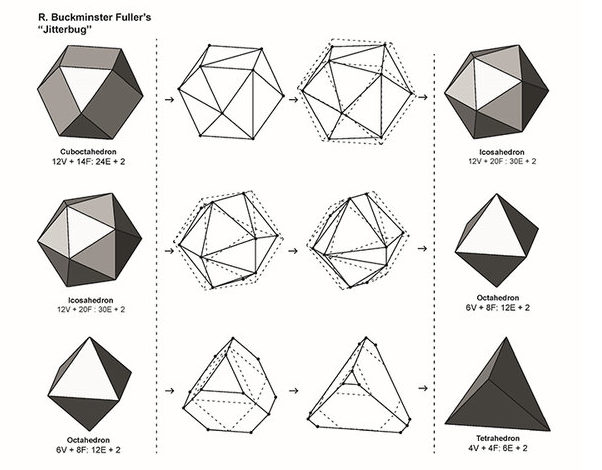“As the VE collapses inward and the square faces contract across one of their diagonals, the length of that diagonal distance becomes the same length as the VE’s edges.  At this moment the symmetry of the icosahedron arises.”15

The dodecahedron is energetically implied at this phase as it is the dual of the icosahedron.

“Continuing on its inward journey, the square faces of the VE continue to contract across the diagonal until the gap is completely closed.  At this moment the symmetry of the octahedron arises.  This octahedral phase now displays a doubling of the vectors of the VE, creating an extremely strong bonding tension as is found in atomic elements that have octahedral symmetry.”16

The cube is implied at this point, as it is the dual of the octahedron.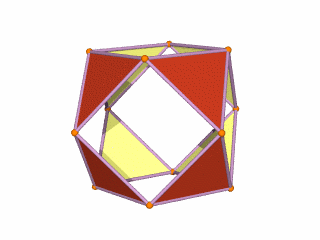From an octahedron, the motion continues, folding in on itself to form the tetrahedron.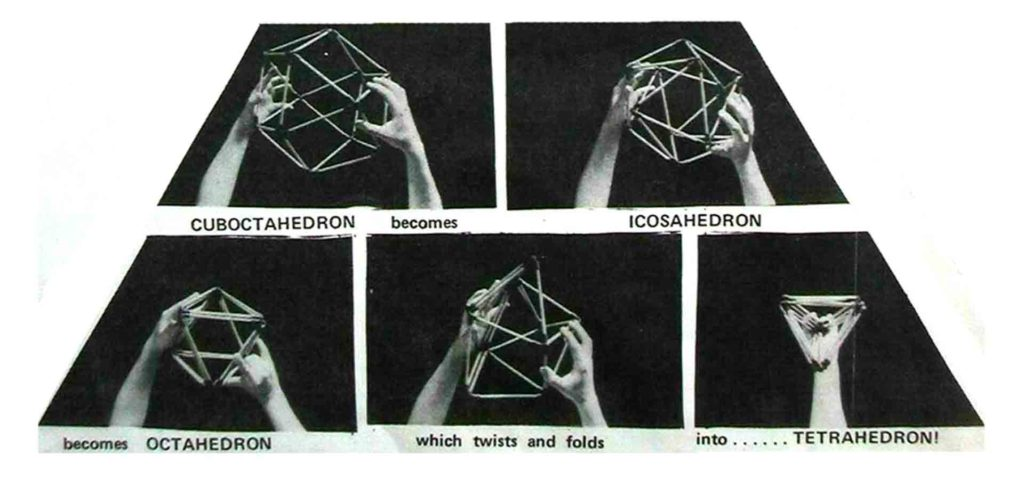Jitterbugging as Toroidal Flow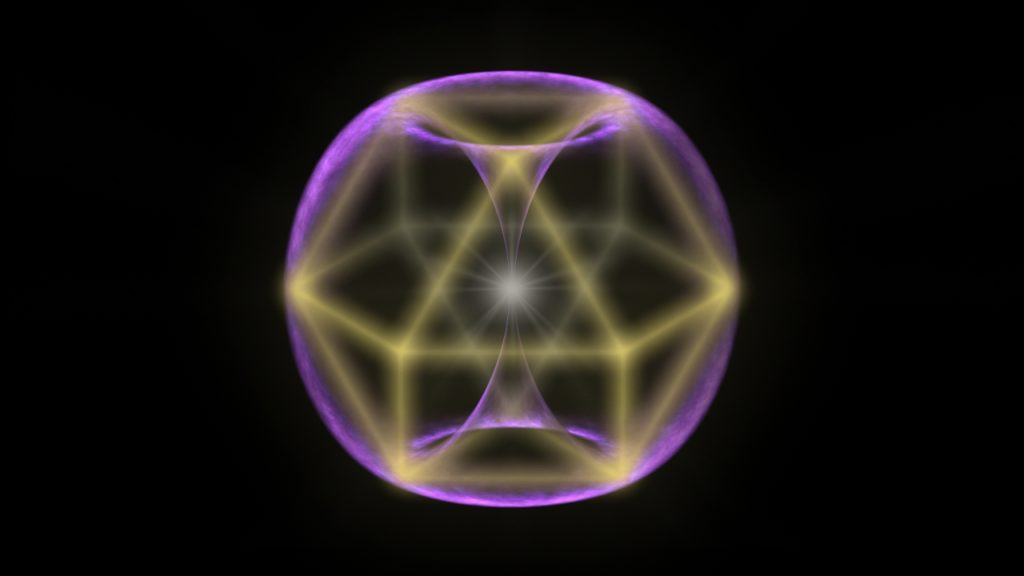“As the VE jitterbug spins inward it sets up a differential of energy density (i.e. pressure, electromagnetic charge) that sets in motion a dual vortex flow that creates the form of a torus. The pumping of the jitterbug sustains this toroidal form in a balanced rhythmic exchange of energy that flows through the manifest system. From a fractal-holographic perspective, it is this fundamental dynamic that takes place at every scale, first expressed as photons, then sub-atomic particles, which then aggregate into the geometric arrays of atoms, which aggregate into compounds that form crystals, minerals, cells and organs, and then whole organisms such as trees, animals, us, and then ecosystems, atmospheres, planets, stars and galaxies.”17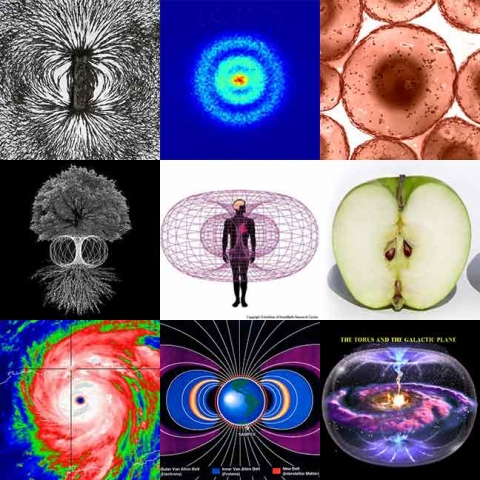1. https://en.wikipedia.org/wiki/Johannes_Kepler
2. ibid.
3. ibid.
4. Gray, Robert, W., Octahedron, Icosahedron, Dodecahedron Triangles, http://www.rwgrayprojects.com/Universe/OctaIcosaDodeca/octaicosadodeca.html
5. https://math.stackexchange.com/questions/1340470/how-to-make-an-icosahedron-from-20-tetrahedra
6. http://www.matematicasvisuales.com/english/html/geometry/space/icosahedron.html
7. https://paulscottinfo.ipage.com/polyhedra/platonic/icosahedron.html
8. Blaze Labs, The Particle: The Wrong Turn that led Physics to a Dead End, http://blazelabs.com/f-p-swave.asp
9. Lawlor, Robert, Sacred Geometry: Philosophy & Practice, Thames & Hudson, 1982
10. ibid.
11. ibid.
12. ibid.
13. ibid.
14. ibid.
15. Lefferts, Marshall, Vector Equilibrium & Isotropic Vector Matrix, http://cosmometry.net/vector-equilibrium-&-isotropic-vector-matrix
16. ibid.
17. ibid.